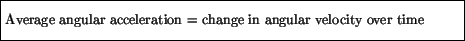Next: Torque Up: Circular motion Previous: Angular velocity

## Angular acceleration

The last analogy with linear motion is the concept of angular acceleration. As with angular velocity, one first introduces the average angular acceleration between two points A and B asand then the instantaneous angular acceleration at A is defined to be the average angular acceleration between A and a point B as the point B approaches A:Angular acceleration is measured in radians / second2.

modtech@theory.uwinnipeg.ca
1999-09-29